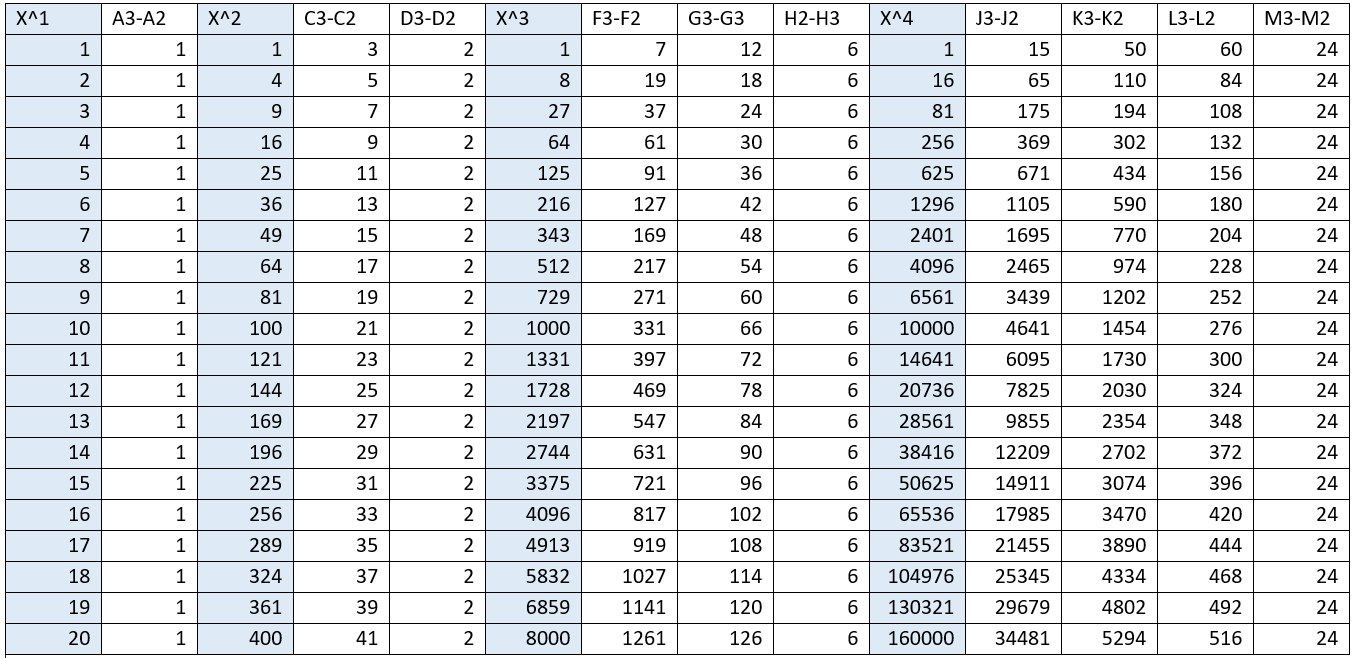# Finding a linear pattern in the powers of integers

I wondered whether I could find a pattern within in the powers of integers. At first sight, it seems like there are no linear differences between the powers. Namely, 2^1=2, 2^2,=4, 2^3=8, 2^4=16, 2^5=32, and so on (you can also look ahead at table 1 to find more examples). In 2^X, the difference between each power is 2,4,8,20 respectively. As you can see in the next picture, the different powers per integer seem to be drifting away from each other. That is no surprise, because we know that 2*2 (4) is way less than 2*2*2 (8). But is there any underlying pattern here?Differences in outcomes per exponent plotted for 1,2,3,4. And exponents ^1,^2,^3,^4. I only plotted these because the difference deviates very much quite quickly

To find a pattern, I took several integers (1-100), (depicted in the rows of table 1), and their powers X^2, X^3, X^4, X^5, and X^6 (highlighted in blue until the power of 4 in table 1). The first thing I noticed when doing this, is that the differences between the outcomes of the power, X^1 is always 1. Aka, the differences between 1,2,3,4,5,6 etc. per integer is equal to 1 (also shown in column (A3-A2) in table 1. This was a clue for me. So I took the outcomes of the power X^2 and calculated the difference, which was 3,5,7,9,11 (column C3-C2). Not the same regularity as x^1, but it was not hard to recognize that the difference from that difference was 2. I went on to X^3, took three times the difference and found the number 6 (H2-H3). I went to X^4, took 4 times the difference and found the number 24. I went too X^5, the five time difference was 120, and with X^6, the sixth time difference a constant of 720 remained.Table 1.

So I found a regularity between the integers of each power when taking the difference according to the amount of powers. I depicted the regular value’s in table 2. In table 2, the sequence seems a bit weird at first. But if you multiply each integer by the exponent of the following integer, you get the following number in the sequence. Namely, 2*3=6, 6*4=24, 24*5=120, and 120*6=720 (there are more ways to do this). So we are back at 1,2,3,4,5,6.

Conclusions
I found a regular pattern within the powers but in quite a weird way. You can find the regularity when you take the amount of differences between the powers over the integers. So with X^1, you take 1 difference (of the outcome), and with X^4 you take four differences (of the outcomes). This gives you a sequence which is regular if you multiply the integer by the power of the following integer. You’ll get 1,2,3,4,5,6 … It was fun to find this. Nevertheless, I don’t know the exact implications of this finding. I can imagine that it becomes easier to find the outcomes of a sequences (using exponents) by using this method. I’m no formal mathematician, so I didn’t put effort in making a formula using this method and applying it. This takes me too much time, given the fact that I’m uncertain about its implications.

If you have any feedback, questions, or know anything about the implications, I would love to hear from you!

Edit:
I later got the following response on “Mymathforum” after posting my blog there to get some feedback, “that the numbers 1, 2, 6, 24, 120, 720, etc., can be written as n! for the nth such number. Thus, 1! = 1, 2! = 2, 3! = 6, etc. By convention, 0! = 1. Note that n! may be read as n factorial. Factorials have lots of interesting properties.”

1.Adam

I have known about this and been examining it off and on in my spare time for years. My teachers and professors always dismissed it as non-significant. There are other interesting properties that you should try to find.

•justintimm